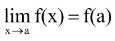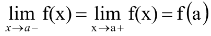# Continuity of A Function

## continuity and differentiability of Class 12

### Definition

A function f(x) is said to be continuous at the point x = a ifi.e.,.

i.e., RHL = LHL = functional value.

The function is said to be continuous in a certain interval [a, b] if f(x) is continuous at every point of the interval. The continuity at boundary points is ascertained by f(b-) = f(b) & f(a) = f(a+).